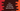# 3 ways to Compare two strings in C++ ignoring case## How to compare two strings in C++ ignoring case:

In this post, we will learn different ways to compare two strings in C++. We will write different C++ programs to compare two strings. It will compare the strings and based on the comparison, it will print one message if both are equal or not.

This comparison will not consider any case, i.e. Hello and hello will be treated as equal strings.

## Method 1: By using ==:

With this method, we will compare two strings by using the comparison operator ==. Below is the complete program:

``````using namespace std;
#include <iostream>
#include <algorithm>

bool compareStrings(string first, string second){
transform(first.begin(), first.end(), first.begin(), ::tolower);
transform(second.begin(), second.end(), second.begin(), ::tolower);

return first == second;
}

int main() {
string firstStr = "Hello World !!";
string secondStr = "hello world !!";
string thirdStr = "hello universe";

if(compareStrings(firstStr, secondStr)){
cout << "firstStr and secondStr are equal"<<endl;
}else{
cout << "firstStr and secondStr are not equal"<<endl;
}

if(compareStrings(firstStr, thirdStr)){
cout << "firstStr and thirdStr are equal"<<endl;
}else{
cout << "firstStr and thirdStr are not equal"<<endl;
}

return 0;
}``````

If you run this program, it will print the below output:

``````firstStr and secondStr are equal
firstStr and thirdStr are not equal``````

Here,

• compareStrings is the method that compares two given strings. It takes two strings first and second as the arguments and returns one boolean value. True if both strings are equal ignoring the character case and False, if both are not equal.
• The main method runs first. Here, we have defined three string variables here.
• The return value of compareStrings method is a boolean. The if-else blocks are checking its return value for different strings. Based on its return value, the if-else blocks prints one message.

## Method 2: By using strncasecmp():

strncasecmp method can be used to compare two strings without case sensitivity. This method takes two strings as the first and the second parameters and a length as the last parameter. The length is the number of characters we want to compare.

This method returns one integer value. If it is less than 0, then the first string is less than the second string. If it is greater than 0, then the first string is greater than the second string. If it is equal to 0, then both strings are equal.

Below is the complete program:

### C++ program:

``````using namespace std;
#include <iostream>
#include <string>
#include <cstring>

bool compareStrings(string first, string second)
{
int first_str_len = first.length();
int second_str_len = second.length();

if (first_str_len != second_str_len)
{
return false;
}

return strncasecmp(first.c_str(), second.c_str(), first_str_len) == 0;
}

int main()
{
string firstStr = "Hello World !!";
string secondStr = "hello world !!";
string thirdStr = "hello universe";

if (compareStrings(firstStr, secondStr))
{
cout << "firstStr and secondStr are equal" << endl;
}
else
{
cout << "firstStr and secondStr are not equal" << endl;
}

if (compareStrings(firstStr, thirdStr))
{
cout << "firstStr and thirdStr are equal" << endl;
}
else
{
cout << "firstStr and thirdStr are not equal" << endl;
}

return 0;
}``````

Here,

• We are using the same strings to compare.
• We need to use cstring header.
• compareStrings method is used to compare two strings. It takes two strings as the parameters. It returns one boolean value.
• It first checks if the length of these strings are equal or not. If both are not equal, it returns false. Else, it uses strncasecmp to compare these strings. This method will be used if the length of both strings are equal. So, we can pass the length of any string as the number of characters to search.

It will print the below output:

``````firstStr and secondStr are equal
firstStr and thirdStr are not equal``````

## Method 3: By using strcasecmp():

strcasecmp can be used to compare strings without any case sensitivity. It takes two strings as its arguments and returns one integer value. This function works only on null terminated strings.

This method returns one integer value. This value is less than 0 if the first string is less than the second string, greater than 0 if the first string is greater than the second string or 0 if both strings are equal.

In our case, we will check if the return value is 0 or not.

### C++ program:

Below is the complete C++ program:

``````using namespace std;
#include <iostream>
#include <string>
#include <cstring>

bool compareStrings(string first, string second)
{
return strcasecmp(first.c_str(), second.c_str()) == 0;
}

int main()
{
string firstStr = "Hello World !!";
string secondStr = "hello world !!";
string thirdStr = "hello universe";

if (compareStrings(firstStr, secondStr))
{
cout << "firstStr and secondStr are equal" << endl;
}
else
{
cout << "firstStr and secondStr are not equal" << endl;
}

if (compareStrings(firstStr, thirdStr))
{
cout << "firstStr and thirdStr are equal" << endl;
}
else
{
cout << "firstStr and thirdStr are not equal" << endl;
}

return 0;
}``````

It will print the same output:

``````firstStr and secondStr are equal
firstStr and thirdStr are not equal``````